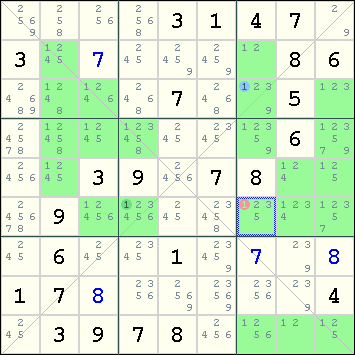Mirrored from Sudopedia, the Free Sudoku Reference Guide

# Crossover

Crossover is a basic technique for Sudoku-X, which says that if there are exactly two cells A and B with candidate X along a diagonal, then X can be eliminated from the other two cells that are seen by both A and B. Crossover can also be applied when there are exactly three cells with the candidate X along the diagonal, as long as one of the cells is r5c5, and the other two cells A and B are located at the same distance away from r5c5.

In other words, the crossover rule is simply Common Peer Elimination applied to the diagonals of the Sudoku-X. When there are exactly two cells along the diagonal having the candidate X, SudoCue replicates crossover using Simple Colors, i.e., a X-Chain containing two cells.

## Example

The following example illustrates the crossover technique.One of the diagonals has exactly two cells having 1 as a candidate: r3c7 and r6c4. Both of these cells see r6c7. Hence, 1 can be eliminated from r6c7.